# Bitwise operators

All our examples we will check by using both binary and decimal numbers show it is better to understand how to convert decimal to Binary and vice versa.
``````#printing decimal to binary
print(bin(120).replace('0b','')) # 1111000``````
``````# binary to decimal
print(int('1111000',2)) # 120``````

## Bitwise AND ( & )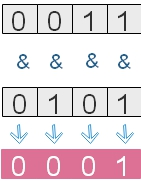Returns 1 when both operands are 1, otherwise returns 0.
``````print(3 & 4) # 0
print(bin(3).replace('0b','')) #  11
print(bin(4).replace('0b','')) # 100

print(12 & 15) # 12
print(bin(12).replace('0b','')) # 1100
print(bin(15).replace('0b','')) # 1111``````

## Bitwise OR ( | )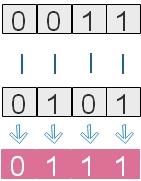Returns 0 if both operands 0, otherwise returns 1.
``````print(12 | 15) # 15
print(bin(12).replace('0b','')) # 1100
print(bin(15).replace('0b','')) # 1111

print(34 | 12) # 46
print(bin(34).replace('0b','')) # 100010
print(bin(12).replace('0b','')) #   1100
print(int('101110',2)) # 46``````

## Bitwise XOR ( ^ )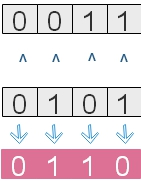Returns 1 of both operands are different, otherwise 0.
``````print(12 ^ 15) # 3
print(bin(12).replace('0b','')) # 1100
print(bin(15).replace('0b','')) # 1111

print(34 ^ 12) #  46
print(bin(34).replace('0b','')) # 100010
print(bin(12).replace('0b','')) #   1100
print(int('101110',2)) #  46``````

## 1's complement (~ )Flips the bits until one 0 is reached (from right side )
This can be represented as
``~x = -x-1 ``
Examples
``````print(~15)  # -16
print(~4)   # -5
print(~-22) # 21
print(~99)  # -100``````

## Bitwise LEFT shift operator ( << )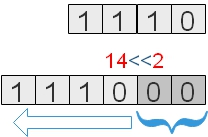This shifts left the binary number by given ( input ) places. The place vacated at left is filled by zeros.
``````print(14<<2)  # 56
print(bin(14).replace('0b','')) #1110``````

## Bitwise RIGHT shift operator ( >> )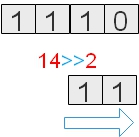This shifts right the binary number by given ( input ) places.
``````print(14>>2)  # 3
print(bin(14).replace('0b','')) #1110

print(90>>3)  # 11
print(bin(90).replace('0b','')) #1011010
print(int('1011',2)) #11``````
1. Examples of Bitwise operator
2. By using Left shift operator by 1 we can multiple the integer by 2
By using Right shift operator by 1 we can divide the integer by 2
``````print(14<<1)   # 28
print(14>>1)   # 7

print(100 <<1) # 200
print(100 >>1) # 50``````
Your Rating

Post your comments , suggestion , error , requirements etc here

We use cookies to improve your browsing experience. . Learn more
 HTML MySQL PHP JavaScript ASP Photoshop Articles FORUM . Contact us
©2000-2019 plus2net.com All rights reserved worldwide Privacy Policy Disclaimer